# OliNo

Renewable Energy

## Line Lite International BV 7W Dimmable Downlight Sharp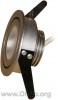presents a 7 W led light bulb that can replace a halogen light bulb. A specific constant current driver was used to make the light measurements. However also a dimmable current driver can be used whit which this liht bulb can be dimmed. Both curent drivers were measured. This article shows the measurement results. Many parameters are also found in the Eulumdat file.

See this overview for a comparison with other light bulbs.

### Summary measurement data

parameter meas. result remark
Color temperature 2918 K Warm white
Luminous intensity Iv 572 Cd
Beam angle 35 An angle well comparable to that of an ordinary halogen light bulb
Power P 9.3 W This is including the constant current driver (not dimmable).
Power Factor 0.58 For every 1 kWh net power consumed, there has been 1.4 kVAhr for reactive power..
Luminous flux 327 lm A lot more than a 35 W halogen light bulb.
Luminous efficacy 35 lm/W
CRI_Ra 74 Color Rendering Index.
Coordinates chromaticity diagram x=0.4357 and y=0.3922
Fitting 230V This light bulb needs a constant current driver that can be conected to the mains voltage.
D x H external dimensions 91 x 39 mm External dimensions of the light bulb.
D luminous area 46 mm Dimensions of the luminous area (used in Eulumdat file). This is equal to the diameter of the front side rippled transparent disk behind which the led is mounted.
form factor recessed
General remarks The ambient temperature during the whole set of measurements was 24-24.5 deg C. The body temperature was between 41 deg C and 51 deg C.
Warm up effect: during the warm up time the illuminance and consumed power both decreased with 7 %.Voltage dependency: the power consumption and illuminance vary less than 4 % when the voltage is varied from 200 – 250 V, hence negligible.This article contains additional photos of the powersupplies used.

This lightbulb is tested on dim-performance.

Measurement report (PDF)Eulumdat fileRight click on icon and save the file.

### Eulumdat light diagram

An interesting graph is the light diagram, indicating the intensity in the C0-C180 and the C90-C270 plane. This light diagram below comes from the program Qlumedit, that extracts these diagrams from an Eulumdat file.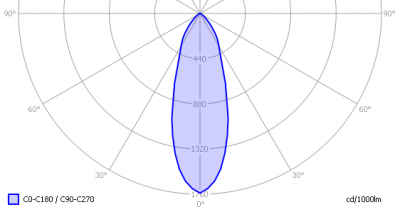The light diagram giving the radiation pattern.

It indicates the luminous intensity around the light bulb. This light bulb has the same light diagram for the C0-C180 as for the C90-C270 plane.

The bundle is comparable to that of an ordinary halogen light bulb.

The unit is Cd/1000lm, meaning the intensity in Cd assuming there would be 1000 lumen in the measured light bulb. This enables comparing different types of light bulbs.

### Illuminance Ev at 1 m distance, or luminous intensity Iv

Herewith the plot of the averaged luminous intensity Iv as a function of the inclination angle with the light bulb.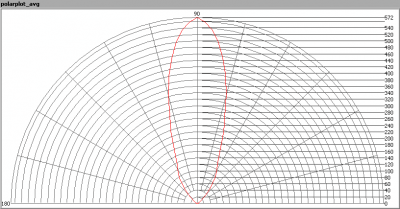The radiation pattern of the light bulb.

This radiation pattern is the average of the light output of the light diagram given earlier. Also, in this graph the luminous intensity is given in Cd.

These averaged values are used (later) to compute the lumen output.Intensity data of every measured turn angle at each inclination angle.

This plot shows per inclination angle the intensity measurement results for each turn angle at that inclination angle. There are differences in illuminance values for different turn angles. However for further calculations the averaged values will be used.

When using the average values per inclination angle, the beam angle can be computed, being 35 degrees. This is the average value but as the light bulb has a symmetry over its z-axis, this angle is valid for all turn-angles the light bulb is looked at.

### Luminous flux

With the averaged illuminance data at 1 m distance, taken from the graph showing the averaged radiation pattern, it is possible to compute the luminous flux.

The result of this computation for this light spot is a luminous flux of 327 lm.

### Luminous efficacy

The luminous flux being 327 lm, and the power of the lightbulb being 9.3 W, yields a luminous efficacy of 35 lm/W.

A power factor of 0.58 means that for every 1 kWh net power consumed, a reactive component of 1.4 kVAr was needed.

 Light bulb voltage (used on power supply!) 230 VAC Light bulb current 70 mA Power P 9.3 W Apparent power S 16.1 VA Power factor 0.58

Of this light bulb the voltage across ad the resulting current through it+its constant current driver power supply are measured and graphed.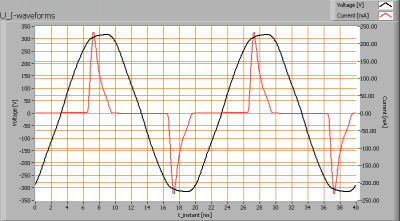Voltage across and current through the lightbulb
There are peaks in current near the tops of the voltage. Also the peaks are not symmetrical with respect to the peaks of the sinuses of the voltage. This results in a power factor less than 1.
Also the power spectrum of the current is determined.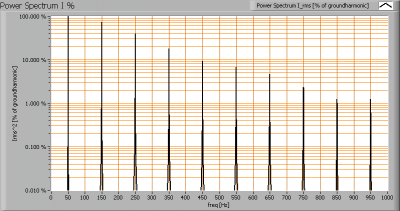Current power spectrum in % of the first harmonic (50 Hz).

The current peaks result in a current power spectrum with a considerable amount of higher harmonics.

### Color temperature and Spectral power distribution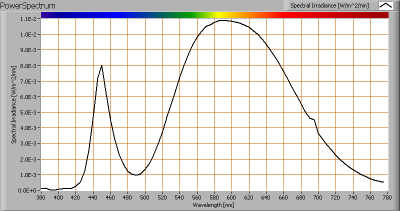The spectral power distribution of this light bulb.

The measured color temperature is about 2900 K which is warm white.

This color temperature is measured straight underneath the light bulb. Below a graph showing the color temperature for different inclination angles.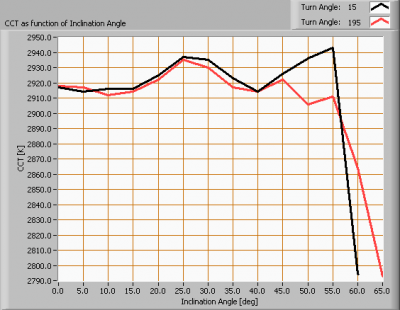Color temperature as a function of inclination angle.

The color temperature is given for inclination angles up to 60 degrees, since at higher angles there is almost no light output anymore.

The value remains constant, for the first 55 degrees, within 1 %. Thereafter there is a quick drop in color temperature.

### Chromaticity diagramThe chromaticity space and the position of the lamp’s color coordinates in it.

The light coming from this lamp is at a distance from the Planckian Locus (the black path in the graph).

Its coordinates are x=0.4357 and y=0.3922.

### Color Rendering Index (CRI) or also Ra

Herewith the image showing the CRI as well as how well different colors are represented (rendered). The higher the number, the better the resemblance with the color when a black body radiator would have been used (the sun, or an incandescent lamp).

Each color has an index Rx, and the first 8 indexes (R1 .. R8) are averaged to compute the Ra which is equivalent to the CRI.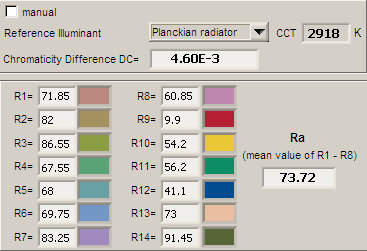CRI of the light of this lightbulb.

The value of 74 is lower than 80 which is considered a minimum value for indoor usage.

Note: the chromaticity difference is 0.0046 indicates the distance to the Planckian Locus. Its value is lower than 0.0054, which means that the calculated CRI result is meaningful.

### Voltage dependency

The dependency of a number of lamp parameters on the lamp voltage is determined. For this, the lamp voltage has been varied and its effect on the following light bulb parameters measured: illuminance E_v [lx], the lamppower P [W], the (Correlated) Color Temperature [K] and the luminous efficacy [lm/W].Lamp voltage dependencies of certain light bulb parameters, where the value at 230 V is taken as 100 %.

The consumed power and illuminance vary < 3 % when the voltage varies between 200-250 V. This variation is insignificant.

When the voltage at 230 V varies with + and – 5 V, then the illuminance varies within 0.25 %, so when abrupt voltage changes occur this effect is not visible in the illuminance output.

### Warm up effects

After switch on of a cold lamp, the effect of heating up of the lamp is measured on illuminance E_v [lx], color temperature CT or correlated color temperature CCT [K], the lamppower P [W] and the luminous efficacy [lm/W].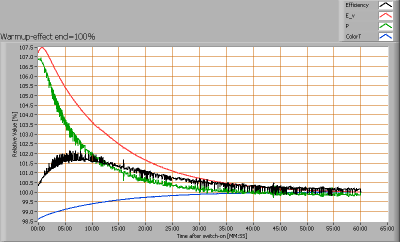Effect of warming up on different light bulb parameters. At top the 100 % level is put at begin, and at bottom at the end.

The warm-up time is about 30 minutes, during which the illumination and consumed power drop about 7 %.

### Dim performance

This lightbulb has been tested on dim-performance. It was connected using the guidelines as given on the website of Line Lite International BV. Also, instead of the constant current driver, a dimmable current driver was used instead.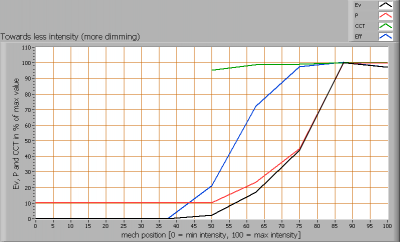Light bulb parameter values depending on the mechanical position of the knob of the dimmer

The light bulb is dimmed using a mechanical range of the knob of about 35 % (from 85 % to 50 % scale). This is enough to well dim the light bulb.

In this range, the light output can be dimmed from 100 % down to 0 %. This is the whole range.

When dimming the light output, the luminous efficacy decreases with increasing dimming level; simply because the amount of energy taken by the dim-unit is relatively more than the amount of energy used by the LEDs themselves.

This graph outputs the parameters when starting at 100 % light output and increasing dimming levels. Going from 0 % light output towards 100 % gives the same result.The used constant current driver (not dimmable).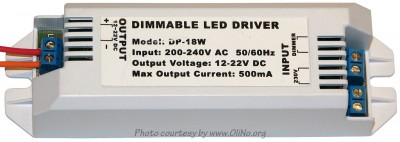The used dimmable current driver.

### Body temperature light bulb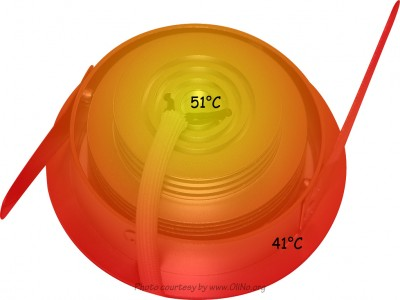Image of the measured body temperature.